# Sample Data: Lansing Woods

Locations of trees in Lansing Woods annotated with species marks

## Details

Locations of trees in Lansing Woods in the observation region Rectangle[{0, 0}, {1, 1}]*924 feet, annoated with species marks.

## Examples

### Basic Examples (1)

 In:=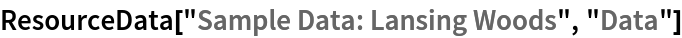Out=Summary of the spatial point data:

 In:=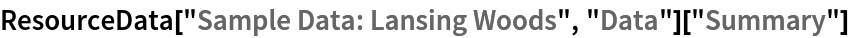Out=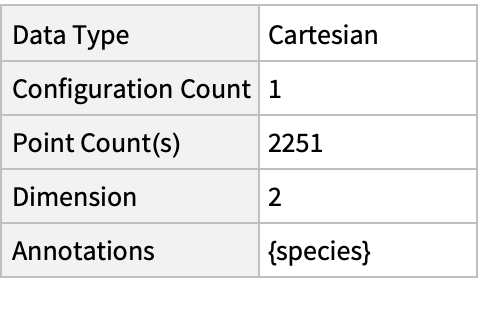### Visualizations (3)

Plot the spatial point data:

 In:=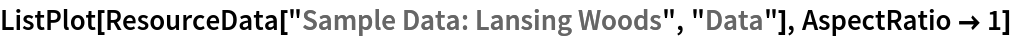Out=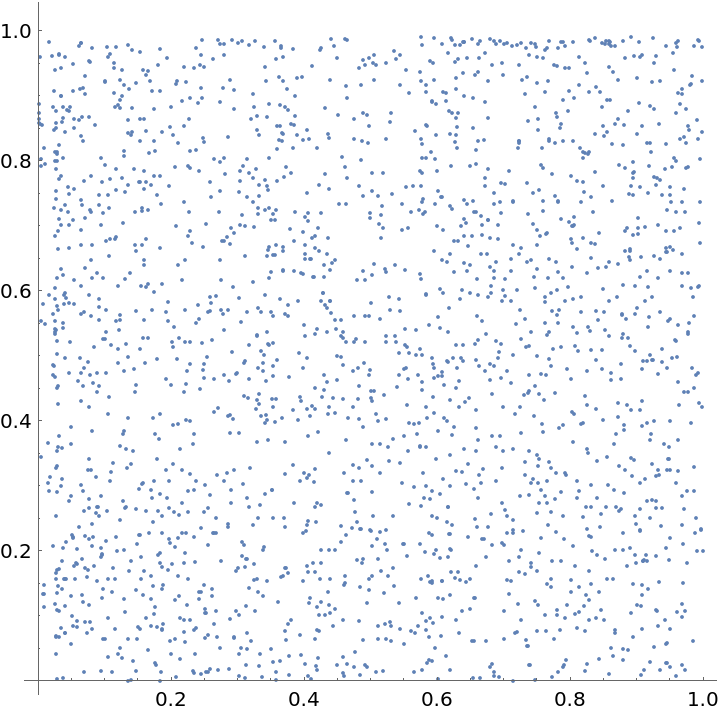Visualize data with annotations:

 In:=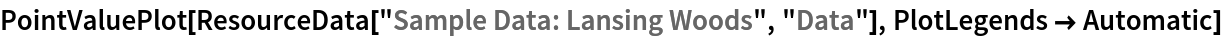Out=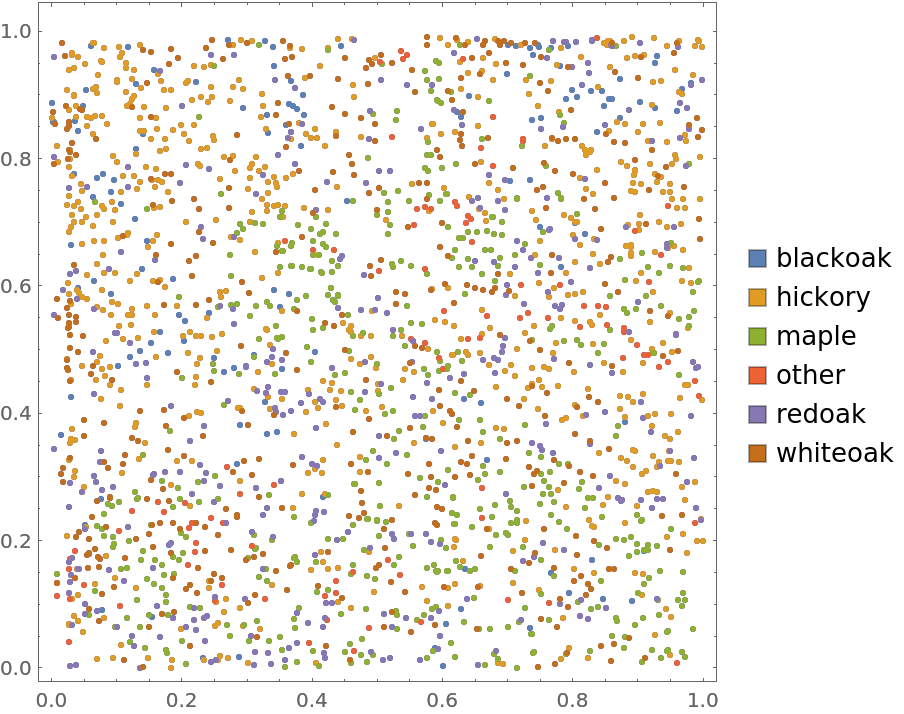Visualize smooth point density:

 In:=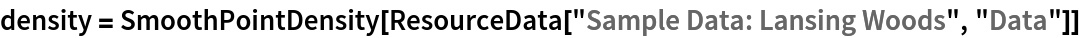Out=In:=Out=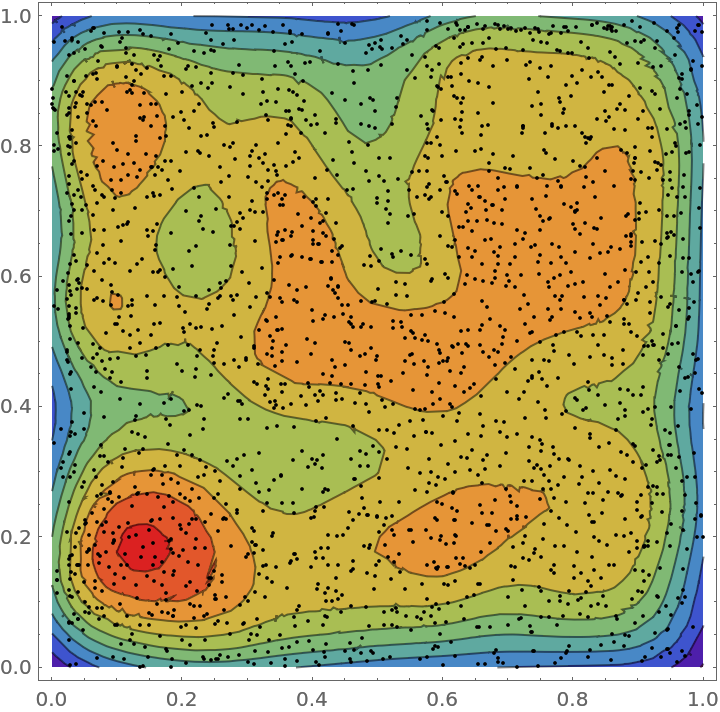### Analysis (4)

Compute probability of finding a point within given radius of an existing point - NearestNeighborG is the CDF of the nearest neighbor distribution:

 In:=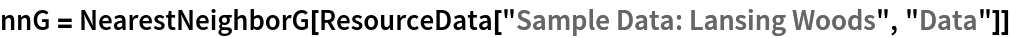Out=In:=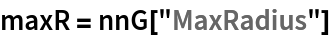Out=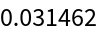In:=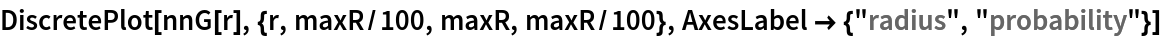Out=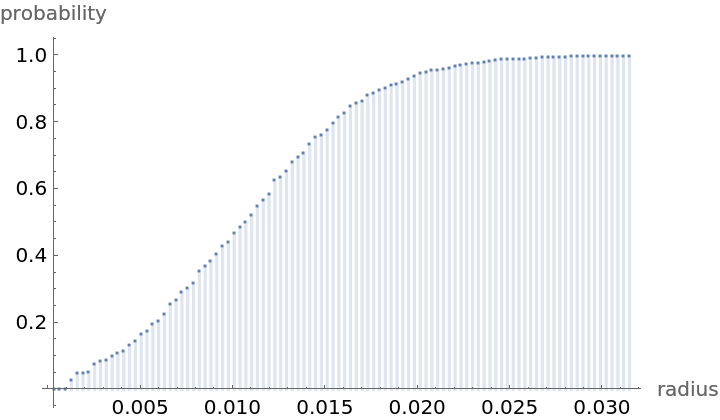Mean distance between a typical point and its nearest neighbor (for positive support distribution can be approximated via a Riemann sum of 1-CDF):

 In:=In:=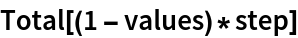Out=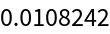Account for scale and units:

 In:=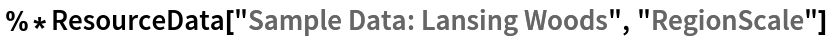Out=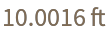Test for complete spacial randomness:

 In:=Out=Gosia Konwerska, "Sample Data: Lansing Woods" from the Wolfram Data Repository (2021)